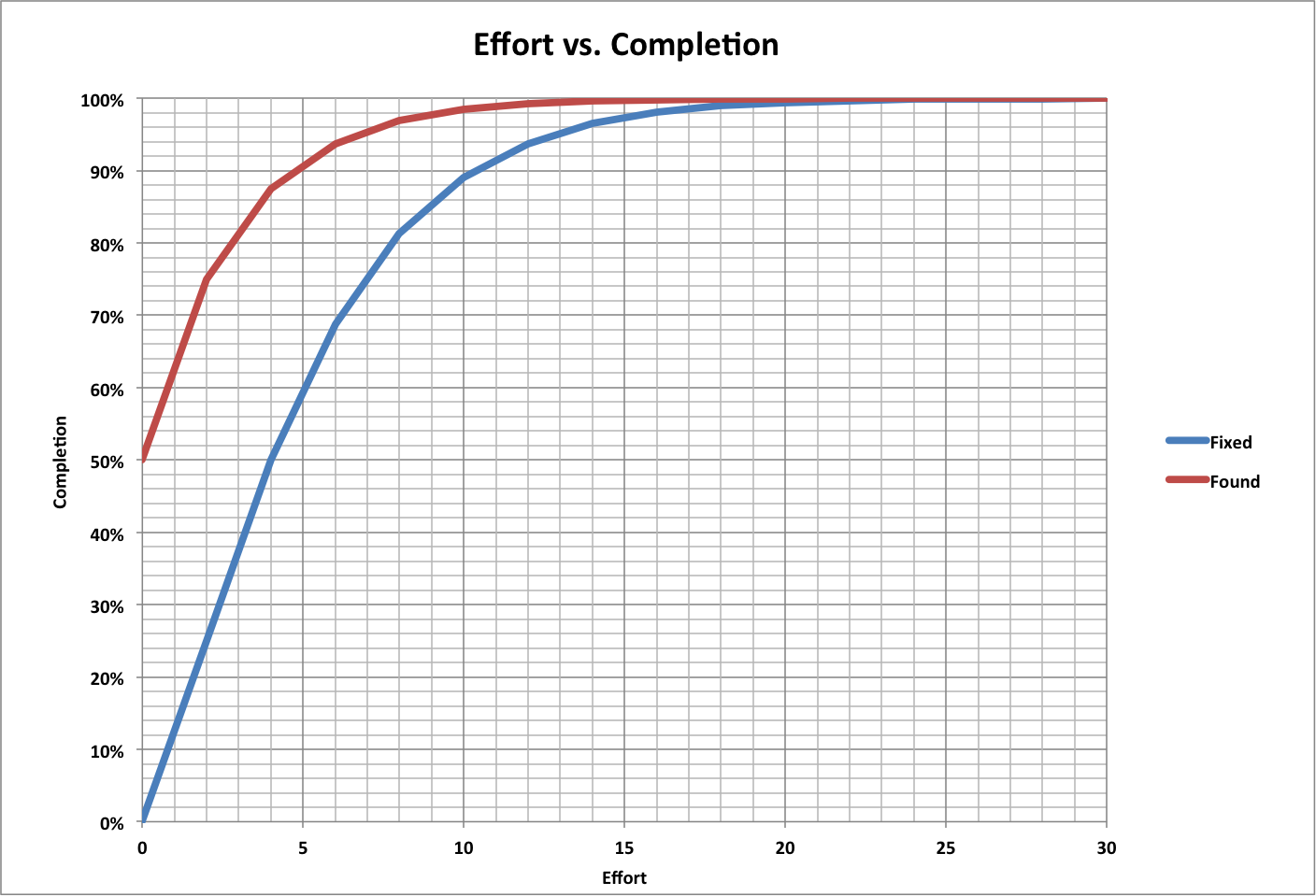# Fixing The Unknown

Fixing a problem, like completion of a task, often assumes that the problem is well-defined (known). In reality there will also be an unknown component of the problem that must be found and then fixed.

Following is a flowchart of this process, which stops when a certain fraction of the problem has been fixed (minimum completion, or "fixed threshold").

Both fixing and finding are done with a degree of efficiency, which determines how much of the remaining task will be completed in an interval of time equal to the ideal needed to complete the task (with 100% eficiency). A typical efficiency is 50% for someone who already has the basic requisite skills.

In this process, two people or groups (depending on the size of the problem) are each providing a unit of "effort" per unit of ideal time, for a total of two units of effort during each interval.The completion as a function of effort for fixing and finding is graphed below, where effort is the total for both (2 / ideal time). Note that the example assumes that the amount of the problem that is unknown is equal to the amount that is known; that is, 1/2 of the problem is known at the beginning of the process.The amount of the problem that is unknown determines how soon as the fixed threshold is reached or exceeded, as shown in the following graph where the amount is represented by the ratio of  the unknown part to the known part. As above, the efficiencies for both fixing and finding are assumed to be 50%, and time is in units of ideal time.

In each case, there are maximum values for time and effort that apply for all higher ratios of unknown to known.The following graph shows how minimum and maximum effort change with the fixed threshold. Note that the maximum effort (most unknown, effort = 2 * time) is 2.2-6 times the minimum effort (everything known, effort = time).Note that for a 99% fixed threshold, the minimum effort is 7 (fixing only) and the maximum effort equals 11 * (FindEffort + 1) where FindEffort is the actual number of people or groups involved in finding the unknown parts of the problem (as a multiple of those fixing the known parts of the problem).

See also:

© Copyright 2016 Bradley Jarvis. All rights reserved.